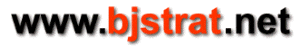## Risk of ruin for fixed wager, fixed EV, and even money payoff

Consider a game in which the player always bets 1 unit. If he wins a bet then his bankroll increases by 1 unit and if he loses it decreases by 1 unit. If he ties then his bankroll remains unchanged. Player decides before he begins playing that he would like to increase his bankroll by a number of units, but he is only willing to risk a set amount of units in trying to achieve his goal.

This calculator mathematically computes the probability of success/failure in such a game given how much player is willing to risk trying to reach a predetermined goal, at which point he'll accept his profit. These amounts are input.

There is one more set of (related) variables that affects player's chances and that is the probability of a win, loss, or tie. Relationship of win, loss, tie (probabilities are expressed in percent) is below:

prob(win in %) + prob(loss in %) + prob(tie in %) = 100%
Expected value (EV in %) = prob(win in %) - prob(loss in %)

Once probability of a tie is input then either EV or win rate can be input to complete the needed information. These 2 inputs are interchangeable and inputting either one automatically updates the value of the other.

This is a purely mathematical calculation. There are no statistical methods involved. However, it is possible that some inputs can result in numbers outside of the range that can be normally interpreted by a computer. In these cases a limiting estimate is computed. This occurs when negative expected value (or win rate less than 50%) is input with a large 'Units to Risk' and/or 'Units Profit For Success'. This is the math theory.

Risk of Ruin Calculator

Sample Input #1 - A gambler is at a 1.41% disadvantage in the game of craps on a pass line bet. On a pass line bet there is no possibility of a tie. Gambler's expected value is -1.41%. It is negative because house has the edge and not the gambler. If gambler had the edge EV would be positive. Assume gambler wants to increase his bankroll by 10 units by playing the pass line in craps but only is willing to risk 10 units. If he wins 10 units he is done and likewise if he loses 10 units he is done. To compute risk of losing 10 units, make these inputs and then click 'Compute Risk of Ruin':

• 'Tie Rate': 0 (0% is default value)
• 'Units to Risk': 10 (10 is default value)
• 'Units Profit For Success': 10 (10 is default value)
• 'Expected Value': -1.41 (which is equivalent to a win rate of 49.295%)

After 'Compute Risk of Ruin' is clicked 57.004% is displayed, which is the probability of losing 10 units before profitting by 10 units.
The probability of profitting by 10 units before losing 10 units is (100% - 57.004% = 42.996%).

Sample Input #2 - A gambler is at a 1.36% disadvantage in the game of craps on a don't pass line bet. On a don't pass line bet there is a possibility of a tie whenever a hard 12 (6-6) is thrown on the first roll of the dice. This happens 1/36 = approximately 2.7778% of the time. Gambler's expected value is -1.36%. It is negative because house has the edge and not the gambler. If gambler had the edge EV would be positive. Assume gambler wants to increase his bankroll by 10 units by playing the don't pass line in craps but only is willing to risk 10 units. If he wins 10 units he is done and likewise if he loses 10 units he is done. To compute risk of losing 10 units, make these inputs and then click 'Compute Risk of Ruin':

• 'Tie Rate': 2.7778 (1/36 = approx 2.7778%)
• 'Units to Risk': 10 (10 is default value)
• 'Units Profit For Success': 10 (10 is default value)
• 'Expected Value': -1.36 (which is equivalent to a win rate of 47.931% with tie rate = 2.7778%)

After 'Compute Risk of Ruin' is clicked 56.949% is displayed, which is the probability of losing 10 units before profitting by 10 units.
The probability of profitting by 10 units before losing 10 units is (100% - 56.949% = 43.051%).